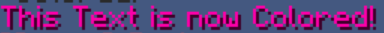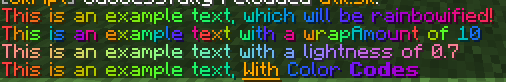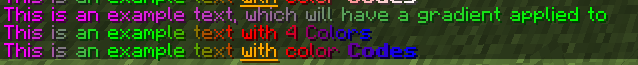# ColorUtils 1.16+

#### Sorbon

VIP
Supporter
Color Utils
By Mr. Darth and Sorbon

12.11.2022 Bugfix MC 1.19.2 / Skript 2.6.3
Skript broky, here fixy
You only need to update the Rainbow function

03.02.2022 Update
Decreased parsing time, increased performance by a little
Please note that the script currently doesn't work with 2.6.1, should be fixed in 2.6.2

What is Color Utils?

With update 1.16 a new feature was added, which allows us to use hex codes for colors.
Color Utils contains two main functions.
- Rainbow
More details are below.

But what are Hex Colors?
A hex triplet is a six-digit, three-byte hexadecimal number used in HTML, CSS, SVG, and other computing applications to represent colors. The bytes represent the red, green and blue components of the color. One byte represents a number in the range 00 to FF (in hexadecimal notation), or 0 to 255 in decimal notation. This represents the least (0) to the most (255) intensity of each of the color components. Thus web colors specify colors in the 24-bit RGB color scheme. The hex triplet is formed by concatenating three bytes in hexadecimal notation, in the following order:

• Byte 1: red value (color type red)
• Byte 2: green value (color type green)
• Byte 3: blue value (color type blue)
For example, consider the color where the red/green/blue values are decimal numbers: red=36, green=104, blue=160 (a grayish-blue color). The decimal numbers 36, 104 and 160 are equivalent to the hexadecimal numbers 24, 68 and A0 respectively. The hex triplet is obtained by concatenating the six hexadecimal digits together, 2468A0 in this example.

If any one of the three color values is less than 10 hex (16 decimal), it must be represented with a leading zero so that the triplet always has exactly six digits. For example, the decimal triplet 4, 8, 16 would be represented by the hex digits 04, 08, 10, forming the hex triplet 040810.

The number of colors that can be represented by this system is 166 or 2563 or 224 = 16,777,216.
tl;dr: Hex codes allow us to use 16 million different Colors. yes, we can finally use brown text color
How does one use Hex Colors?
Skript v2.6 allows us to use "<##FF00AA>" for the Hex colors.
The second # in the code is optional, but sometimes you need it, because skript isn't the smartest ;D

Note: If you're using the hex codes syntax in 'send %text%' , you will have to add "formatted" in front of it.
Example:
Code:
``send formatted "<##FF00AA>This Text is now Colored!"``Color Utils Functions:

Patter: RGBToHex(number: numbers)
Return Value: String / Text

Description: Function to convert decimal numbers in the range [0; 255] to their hexadecimal representation.
Parameter:
number: the numbers to be converted to hexadecimal

Code:
``````function RGBToHex(number: numbers) :: string:
loop {_number::*}:
set {_r} to join {_r}, (character at (mod((floor(min(max(loop-value, 0), 255) / 16)), 16) + 1) in "0123456789ABCDEF") and (character at ((mod(min(max(loop-value, 0), 255), 16)) + 1) in "0123456789ABCDEF")
return colored "<##%{_r}%>"``````

Patter: HexToRGB(hex: string)
Return Value: numbers

Description: Function to convert hex to RGB values
Parameter:
Hex: hex value to be converted

Code:
``````function HexToRGB(hex: string) :: numbers:
replace all "##" with "" in {_hex}
set {_s::*} to split "0123456789abcdefklmnor" at ""
loop 3 times:
set {_n} to subtext of {_hex} from characters (loop-value -1)*2+1 to loop-value*2 in lower case
add (index of first element out of (split {_n} at "") in "0123456789abcdefklmnor" -1)* 16 to {_rgb::%loop-value-1%}
add index of 2nd element out of (split {_n} at "") in "0123456789abcdefklmnor"-1  to {_rgb::%loop-value-1%}
return {_rgb::*}``````

Patter: rainbow(input: string, wrapAmount: number, lightness: number)
Return Value: String / Text

Description: Function that colors the string in a rainbow pattern
Parameter:
Input: the string to be rainbowified
WrapAmount: the rainbow pattern will start repeating approximately every wrapAmount characters; Default value is -1
Lightness: a number from 0 to 1 (0% - 100%) representing how light the color should be; a value of 0.5 means no change, 1 is white, 0 is black. Default Value is 0.5

Code:
``````function rainbow(input: string, wrapAmount: number = -1, lightness: number = 0.5) :: string:
set {_delta} to 360 / ({_wrapAmount} if {_wrapAmount} > 0, else length of {_input})
set {_lightnessModifier} to (({_lightness}*2)-1) * 255
set {_angle} to 90
loop length of {_input} times:
set {_character} to character at loop-value in {_input}
if {_character} or (character at (loop-value - 1) in {_input}) is "§":
if "abcdefklmnor0123456789" contains {_character}:
set {_format} to (join {_format} and "&%{_character}%") if {_character} is not "r", else ("")
continue
loop 3 times:
set {_n::%loop-value-2%} to (0.5 * (sin(({_angle} + (loop-number-2*120-120))) + 1)) * 255 + {_lightnessModifier}
set {_output} to join {_output}, RGBToHex({_n::*}), {_format} and {_character}
remove {_delta} from {_angle}
return colored {_output}``````

Code:
``````command example:
trigger:
send formatted rainbow("This is an example text, which will be rainbowified!")
send formatted rainbow("This is an example text with a wrapAmount of 10", 10)
send formatted rainbow("This is an example text with a lightness of 0.7", -1, 0.7)
send formatted rainbow("This is an example text, &6&nWith&r Color &lCodes")``````Return Value: String / Text

Description: Function that applies a gradient pattern over a given string
Parameter:
input: the string to be colored
color: list of colors in HEX (#000000 - #FFFFFF)

Code:
``````function gradient(input: string, color: strings) :: string:
loop {_color::*}:
set {_rgb::%loop-index%::*} to HexToRGB(loop-value)
set {_n} to size of {_color::*} -1
set {_delta} to 180 / (length of uncolored {_input}/{_n} - 1)
loop {_n} times:
loop ceil(length of uncolored {_input}/{_n}) times:
set {_y} to ((loop-value-1) -1)*(ceil((length of uncolored {_input})/{_n})) + (loop-value-2)
while character at {_y}+{_x} in {_input} is "§":
if "abcdefklmnor0123456789" contains character at {_y}+{_x}+1 in {_input}:
set {_format} to (join {_format} and "&%character at {_y}+{_x}+1 in {_input}%") if character at {_y}+{_x}+1 in {_input} is not "r", else ("")
set {_character} to character at {_y} in uncolored {_input}
set {_startColorProportion} to (0.5 * (sin(({_delta}*((loop-value-2)-1))+90) + 1))
set {_endColorProportion} to (0.5 * (sin(({_delta}*((loop-value-2)-1))+270) + 1))
loop 3 times:
set {_n::%loop-value-3%} to ({_rgb::%loop-value-1%::%loop-value-3%} * {_startColorProportion}) + ({_rgb::%((loop-value-1) + 1)%::%loop-value-3%} * {_endColorProportion})
set {_output} to join {_output}, RGBToHex({_n::*}), {_format} and {_character}
return colored {_output}``````

Code:
``````command example:
trigger:
send formatted gradient("This is an example text, which will have a gradient applied to", ("FF00FF", "00FF00"))
send formatted gradient("This is an example text with 4 Colors", ("FF00FF", "00FF00","FF0000", "0000FF"))
send formatted gradient("This is an example text &6&nwith&r color &lCodes", ("FF00FF", "00FF00","FF0000", "0000FF"))``````Code:
``````#    _____      _              _    _ _   _ _
#   / ____|    | |            | |  | | | (_) |
#  | |     ___ | | ___  _ __  | |  | | |_ _| |___ TM    | A colorful script brought to you by Sourbun et Mr. Darth :D
#  | |    / _ \| |/ _ \| '__| | |  | | __| | / __|      | Version 2.3
#  | |___| (_) | | (_) | |    | |__| | |_| | \__ \
#   \_____\___/|_|\___/|_|     \____/ \__|_|_|___/

# A small set of functions designed to bring a new look to your server, giving it color :)
# Nota Bene: These functions are pretty fast, yet using them a lot of times in a short period of time will probably cause quite some lag.

# Update 2.3: Decreased Parsing time and increased performance

# RGBToHex(number: numbers)
# @description Function converts RGB Value to the HEX representation
# @param number: list of numbers between 0 and 255
# @return the hexadecimal representation of the numbers
function RGBToHex(number: numbers) :: string:
loop {_number::*}:
set {_r} to join {_r}, (character at (mod((floor(min(max(loop-value, 0), 255) / 16)), 16) + 1) in "0123456789ABCDEF") and (character at ((mod(min(max(loop-value, 0), 255), 16)) + 1) in "0123456789ABCDEF")
return colored "<##%{_r}%>"

#HexToRGB(hex: string)
# @description Function to convert a hex value to the 3 Color values
# @param: 6 letter hex string
# @return 3 numbers
function HexToRGB(hex: string) :: numbers:
replace all "##" with "" in {_hex}
set {_s::*} to split "0123456789abcdefklmnor" at ""
loop 3 times:
set {_n} to subtext of {_hex} from characters (loop-value -1)*2+1 to loop-value*2 in lower case
add (index of first element out of (split {_n} at "") in "0123456789abcdefklmnor" -1)* 16 to {_rgb::%loop-value-1%}
add index of 2nd element out of (split {_n} at "") in "0123456789abcdefklmnor"-1  to {_rgb::%loop-value-1%}
return {_rgb::*}

# rainbow(input: string, wrap: boolean = false, wrapAmount: number = 10, lightness: number = 0)
# @description Function that 'rainbowifies' a given string (id est colors the string in a rainbow pattern - ROYGBIV)
# @param input the string to be rainbowified
# @param wrapAmount if this value is positive, the rainbow pattern will start repeating approximately every wrapAmount characters
# @param lightness a number from 0 to 1 representing how light the color should be; a value of 0.5 means no change, value 0 is black and value 1 is white
# @return the rainbowified string
function rainbow(input: string, wrapAmount: number = -1, lightness: number = 0.5) :: string:
set {_delta} to 360 / ({_wrapAmount} if {_wrapAmount} > 0, else length of {_input})
set {_lightnessModifier} to (({_lightness}*2)-1) * 255
set {_angle} to 90
loop length of {_input} times:
set {_character} to character at loop-value in {_input}
if {_character} or (character at (loop-value - 1) in {_input}) is "§":
if "abcdefklmnor0123456789" contains {_character}:
set {_format} to (join {_format} and "&%{_character}%") if {_character} is not "r", else ("")
continue
loop 3 times:
set {_n::%loop-value-2%} to (0.5 * (sin(({_angle} + (loop-number-2*120-120))) + 1)) * 255 + {_lightnessModifier}
set {_output} to join {_output}, RGBToHex({_n::*}), {_format} and {_character}
remove {_delta} from {_angle}
return colored {_output}

# gradient(input: string, startColor: numbers, endColor: numbers)
# @description Function that applies a gradient pattern over a given string
# @param input the string to be colored
# @param color list of Hex colors
# @return the colored string in a gradient pattern
function gradient(input: string, color: strings) :: string:
loop {_color::*}:
set {_rgb::%loop-index%::*} to HexToRGB(loop-value)
set {_n} to size of {_color::*} -1
set {_delta} to 180 / (length of uncolored {_input}/{_n} - 1)
loop {_n} times:
loop ceil(length of uncolored {_input}/{_n}) times:
set {_y} to ((loop-value-1) -1)*(ceil((length of uncolored {_input})/{_n})) + (loop-value-2)
while character at {_y}+{_x} in {_input} is "§":
if "abcdefklmnor0123456789" contains character at {_y}+{_x}+1 in {_input}:
set {_format} to (join {_format} and "&%character at {_y}+{_x}+1 in {_input}%") if character at {_y}+{_x}+1 in {_input} is not "r", else ("")
set {_character} to character at {_y} in uncolored {_input}
set {_startColorProportion} to (0.5 * (sin(({_delta}*((loop-value-2)-1))+90) + 1))
set {_endColorProportion} to (0.5 * (sin(({_delta}*((loop-value-2)-1))+270) + 1))
loop 3 times:
set {_n::%loop-value-3%} to ({_rgb::%loop-value-1%::%loop-value-3%} * {_startColorProportion}) + ({_rgb::%((loop-value-1) + 1)%::%loop-value-3%} * {_endColorProportion})
set {_output} to join {_output}, RGBToHex({_n::*}), {_format} and {_character}
return colored {_output}``````
Code:
``````command exampleRainbow:
trigger:
send formatted rainbow("This is an example text, which will be rainbowified!")
send formatted rainbow("This is an example text with a wrapAmount of 10", 10)
send formatted rainbow("This is an example text with a lightness of 0.7", -1, 0.7)
send formatted rainbow("This is an example text, &6&nWith&r Color &lCodes")

trigger:
send formatted gradient("This is an example text, which will have a gradient applied to", ("FF00FF", "00FF00"))
send formatted gradient("This is an example text with 4 Colors", ("FF00FF", "00FF00","FF0000", "0000FF"))
send formatted gradient("This is an example text &6&nwith&r color &lCodes", ("FF00FF", "00FF00","FF0000", "0000FF"))

on chat:
set message to rainbow(colored message, 20, 0.7)``````Last edited:
•Jande, tcrnado, Ankoki and 2 others
THIS IS AMAZING.

this deserves a pog champ c:

•Mr. Darth
Pog person

Wow!

is there a way to get items with custom colours

is there a way to get items with custom colours
Set name of player's tool to rainbow(name of player's tool)

creator's a cool guy